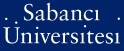# Valid inequalities and restrictions for stochastic programming problems with first order stochastic dominance constraints

Noyan, Nilay and Ruszczynski, Andrzej (2008) Valid inequalities and restrictions for stochastic programming problems with first order stochastic dominance constraints. Mathematical programming, 114 (2). pp. 249-275. ISSN 0025-5610 (Print) 1436-4646 (Online)PDF - Repository staff only - Requires a PDF viewer such as GSview, Xpdf or Adobe Acrobat Reader354Kb

Official URL: http://dx.doi.org/10.1007/s10107-007-0100-1

## Abstract

Stochastic dominance relations are well-studied in statistics, decision theory and economics. Recently, there has been significant interest in introducing dominance relations into stochastic optimization problems as constraints. In the discrete case, stochastic optimization models involving second order stochastic dominance (SSD) constraints can be solved by linear programming (LP). However, problems involving first order stochastic dominance (FSD) constraints are potentially hard due to the non-convexity of the associated feasible regions. In this paper we consider a mixed 01 linear programming formulation of a discrete FSD-constrained optimization model and present an LP relaxation based on SSD constraints. We derive some valid inequalities and restrictions by employing the probabilistic structure of the problem. We also generate cuts that are valid inequalities for the disjunctive relaxations arising from the underlying combinatorial structure of the problem by applying the lift-and-project procedure. We describe three heuristic algorithms to construct feasible solutions, based on conditional SSD-constraints, variable fixing, and conditional value at risk. Finally, we present numerical results for several instances of a portfolio optimization problem.

Item Type: Article stochastic programming; stochastic dominance; valid inequalities; disjunctive cuts; conditional value at risk Q Science > Q Science (General)T Technology > T Technology (General)Q Science > QA Mathematics 234 Nilay Noyan 17 Feb 2007 02:00 17 Sep 2019 14:07

Repository Staff Only: item control page